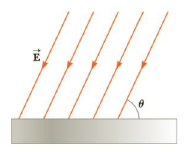Chapter 15, Problem 44P

Chapter
Section
Textbook Problem

A uniform electric field of magnitude E = 435 N/C makes an angle of θ = 65.0° with a plane surface of area A = 3.50 m2 as in Figure P15.44. Find the electric flux through this surface.Figure P15.44

To determine
The electric flux.

Explanation

Given info: The electric field (E) is 435 N/C. The area of the surface (A) is 3.5m2 .

The angle between the electric field and area vector is,

θ=90.0ο65.0ο=25ο

Formula to calculate the electric flux is,

ϕE=EAcosθ

Substitute 435 N/C for E, 3.5m2 for A and 25ο for θ

Still sussing out bartleby?

Check out a sample textbook solution.

See a sample solution

The Solution to Your Study Problems

Bartleby provides explanations to thousands of textbook problems written by our experts, many with advanced degrees!

Get Started

Find more solutions based on key concepts